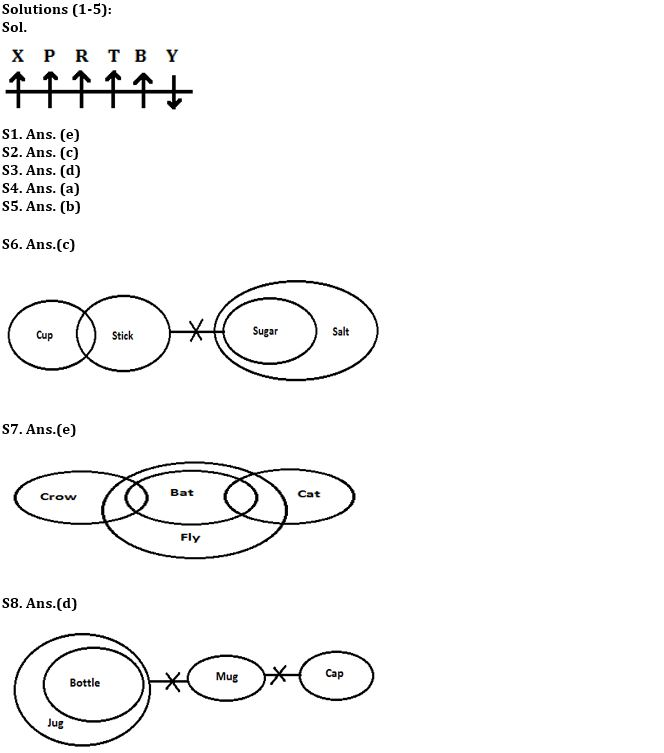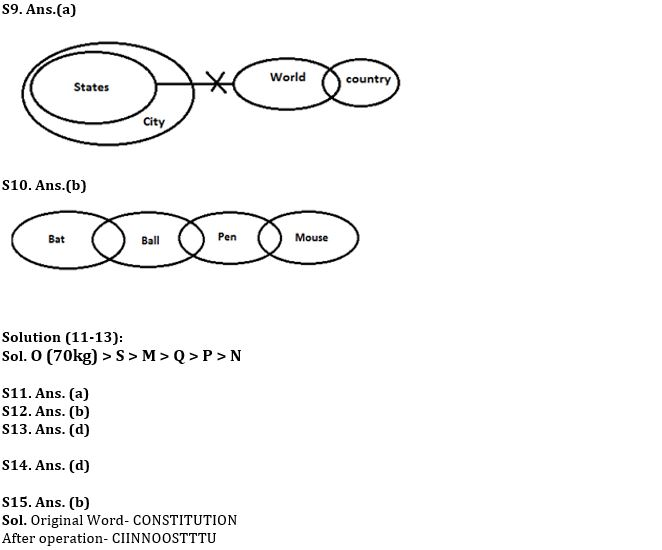Latest Banking jobs   »

# Reasoning Ability Quiz For IBPS Clerk Prelims 2022- 7th August

Directions (1-5): Study the following information carefully and answer the questions given below:
Six persons sit in a row. Some of them are facing to the north while rest are facing to South. P sits 3rd to the left of B and both does not sit at the extreme ends. The persons who sit at the extreme ends are facing opposite direction. There are two persons sit between X and T. X does not sit near to B. R sits 3rd to the right of Y who is an immediate neighbor of B. R faces to the north. Y faces opposite direction with respect to R. Both P and T are facing in the same direction as R.

Q1. How many persons are facing to the north?
(a) Two
(b) Three
(c) One
(d) Four
(e) Five

Q2. Four of the following five are alike in a certain way and hence they form a group. Which one of the following does not belong to that group?
(a) P
(b) T
(c) Y
(d) B
(e) R

Q3. What is the position of X with respect to R?
(a) 3rd to the left
(b) 2nd to the right
(c) Immediate to the left
(d) 2nd to the left
(e) None of these

Q4. How many persons sit right side from T?
(a) Two
(b) One
(c) Three
(d) Four
(e) None of these

Q5. Who among the following persons are sitting at extreme end?
(a) P-X
(b) X-Y
(c) P-T
(d) B-X
(e) R-Y

Directions (6-10): In each question below, some statements are given followed by some conclusions. You have to take the given statements to be true even if they seem to be at variance with commonly known facts. Read all the conclusions and then decide which of the given conclusions logically follows from the given statements, disregarding commonly known facts.
(a) if both conclusion I and II are true.
(b) if neither conclusion I nor II is true.
(c) if only conclusion I is true.
(d) if either conclusion I or II is true.
(e) if only conclusion II is true.

Q6. Statements: All sugar is salt.
Some cup are stick.
No stick is sugar.
Conclusions: I. All cup are salt is a possibility.
II. Some stick are salt.

Q7. Statements: Some crow is bat.
Some bat are cat.
All bat are fly.
Conclusions: I. No fly is cat.
II. Some crow can be cat.

Q8. Statements: All bottle are jug.
No jug is mug.
No mug are cap.
Conclusions: I. Some bottle are cap.
II. No cap are bottle.

Q9. Statements: All states are city.
Some country are world.
No world is states.
Conclusions: I. Some city are states.
II. All states are country is a possibility.

Q10. Statements: Some bat are ball.
Some pen are mouse.
Some ball are pen.
Conclusions: I. Some bat are pen.
II. Some ball are mouse.

Directions (11-13): Study the information carefully and answer the questions given below.
Six persons M, N, O, P, Q, and S are of different weight. P is heavier than N. M is lighter than S. Q is heavier than N. M is not lighter than P and Q. S is not heaviest, and Q is not second lightest. The weight of heaviest is 70kg.

Q11. Who among the following person is third heaviest?
(a) M
(b) S
(c) P
(d) Q
(e) Can’t be determined

Q12. Who among the following is the lightest person?
(a) M
(b) N
(c) Q
(d) P
(e) None of these

Q13. If weight of M is 67kg what may be the weight of S?
(a) 60kg
(b) 63kg
(c) 61kg
(d) 69kg
(e) None of these

Q14. If in the number 39475132, 2 is multiply to each of the digit which is less than 4 and 3 is subtracted from each of the digit which is more than 4 and equal to 4 then how many digits are repeating in the number thus formed?
(a) None
(b) Four
(c) One
(d) Three
(e) Two

Q15. If all the letters in the word CONSTITUTION are arranged in alphabetical order from left to right, then how many alphabets remains same in their position?
(a) Three
(b) One
(c) None
(d) Two
(e) four

Solutions#### Congratulations!Download Hindu Review of October 2021: Free PDF